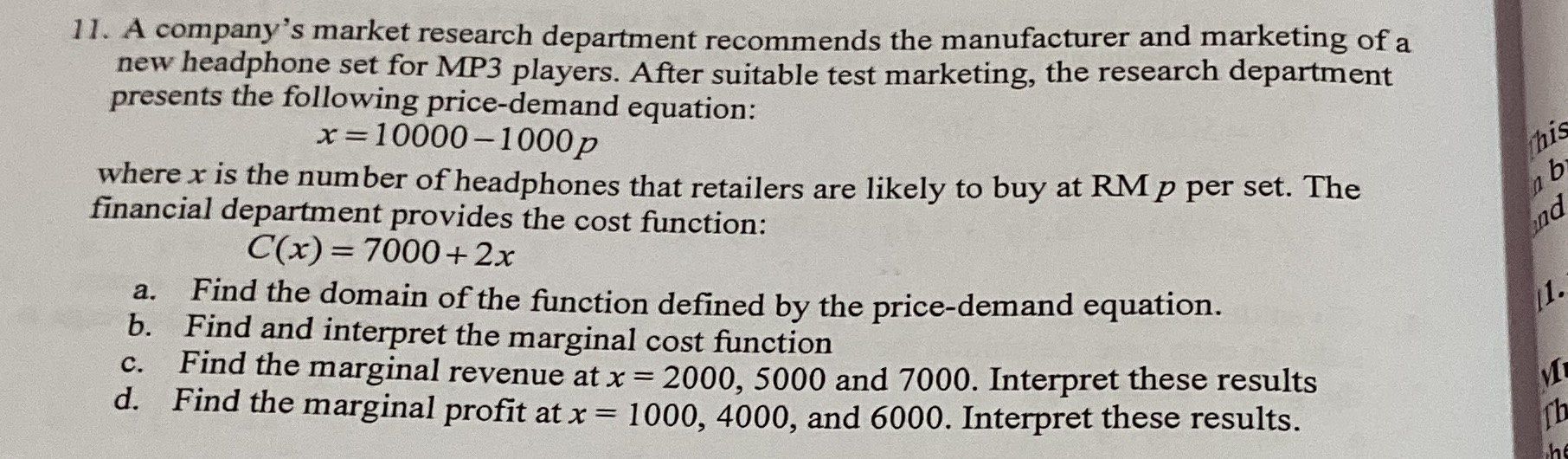### ¿Todavía tienes preguntas de matemáticas?

Pregunte a nuestros tutores expertos
Algebra
Pregunta11. A company's market research department recommends the manufacturer and marketing of a new headphone set for MP3 players. After suitable test marketing, the research department presents the following price-demand equation:

$$x = 10000 - 1000 p$$

where $$x$$ is the number of headphones that retailers are likely to buy at RM $$p$$ per set. The financial department provides the cost function.

$$C ( x ) = 7000 + 2 x$$

a. Find the domain of the function defined by the price-demand equation. b. Find and interpret the marginal cost function c. Find the marginal revenue at $$x = 2000,5000$$ and $$7000$$ . Interpret these results d. Find the marginal profit at $$x = 1000,4000$$ , and $$6000$$ . Interpret these results.